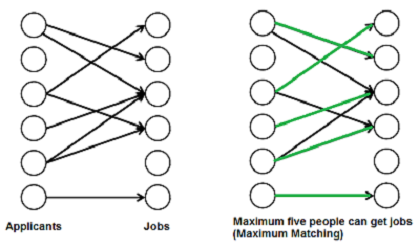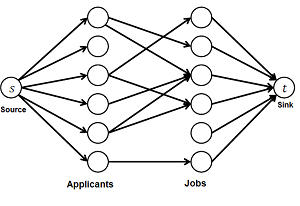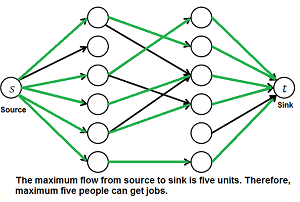# Maximum Bipartite Matching

A matching in a Bipartite Graph is a set of the edges chosen in such a way that no two edges share an endpoint. A maximum matching is a matching of maximum size (maximum number of edges). In a maximum matching, if any edge is added to it, it is no longer a matching. There can be more than one maximum matchings for a given Bipartite Graph.

Why do we care?
There are many real world problems that can be formed as Bipartite Matching. For example, consider the following problem:

There are M job applicants and N jobs. Each applicant has a subset of jobs that he/she is interested in. Each job opening can only accept one applicant and a job applicant can be appointed for only one job. Find an assignment of jobs to applicants in such that as many applicants as possible get jobs.”We strongly recommend to read the following post first. “Ford-Fulkerson Algorithm for Maximum Flow Problem”
Maximum Bipartite Matching and Max Flow Problem :

Maximum Bipartite Matching (MBP) problem can be solved by converting it into a flow network (See this video to know how did we arrive this conclusion). Following are the steps.1) Build a Flow Network : There must be a source and sink in a flow network. So we add a source and add edges from source to all applicants. Similarly, add edges from all jobs to sink. The capacity of every edge is marked as 1 unit.2) Find the maximum flow: We use Ford-Fulkerson algorithm to find the maximum flow in the flow network built in step 1. The maximum flow is actually the MBP we are looking for.

How to implement the above approach?

Let us first define input and output forms. Input is in the form of Edmonds matrix which is a 2D array ‘bpGraph[M][N]’ with M rows (for M job applicants) and N columns (for N jobs). The value bpGraph[i][j] is 1 if i’th applicant is interested in j’th job, otherwise 0.

Output is number maximum number of people that can get jobs.

A simple way to implement this is to create a matrix that represents adjacency matrix representation of a directed graph with M+N+2 vertices. Call the fordFulkerson() for the matrix. This implementation requires O((M+N)*(M+N)) extra space.

Extra space can be reduced and code can be simplified using the fact that the graph is bipartite and capacity of every edge is either 0 or 1. The idea is to use DFS traversal to find a job for an applicant (similar to augmenting path in Ford-Fulkerson). We call bpm() for every applicant, bpm() is the DFS based function that tries all possibilities to assign a job to the applicant.

In bpm(), we one by one try all jobs that an applicant ‘u’ is interested in until we find a job, or all jobs are tried without luck. For every job we try, we do following.

If a job is not assigned to anybody, we simply assign it to the applicant and return true. If a job is assigned to somebody else say x, then we recursively check whether x can be assigned some other job. To make sure that x doesn’t get the same job again, we mark the job ‘v’ as seen before we make recursive call for x. If x can get other job, we change the applicant for job ‘v’ and return true. We use an array maxR[0..N-1] that stores the applicants assigned to different jobs.

If bmp() returns true, then it means that there is an augmenting path in flow network and 1 unit of flow is added to the result in maxBPM().

Recommended Practice

Implementation:

## C++

 `// A C++ program to find maximal` `// Bipartite matching.` `#include ` `#include ` `using` `namespace` `std;`   `// M is number of applicants ` `// and N is number of jobs` `#define M 6` `#define N 6`   `// A DFS based recursive function ` `// that returns true if a matching` `// for vertex u is possible` `bool` `bpm(``bool` `bpGraph[M][N], ``int` `u,` `         ``bool` `seen[], ``int` `matchR[])` `{` `    ``// Try every job one by one` `    ``for` `(``int` `v = 0; v < N; v++)` `    ``{` `        ``// If applicant u is interested in ` `        ``// job v and v is not visited` `        ``if` `(bpGraph[u][v] && !seen[v])` `        ``{` `            ``// Mark v as visited` `            ``seen[v] = ``true``; `   `            ``// If job 'v' is not assigned to an ` `            ``// applicant OR previously assigned ` `            ``// applicant for job v (which is matchR[v]) ` `            ``// has an alternate job available. ` `            ``// Since v is marked as visited in ` `            ``// the above line, matchR[v] in the following ` `            ``// recursive call will not get job 'v' again` `            ``if` `(matchR[v] < 0 || bpm(bpGraph, matchR[v],` `                                     ``seen, matchR))` `            ``{` `                ``matchR[v] = u;` `                ``return` `true``;` `            ``}` `        ``}` `    ``}` `    ``return` `false``;` `}`   `// Returns maximum number` `// of matching from M to N` `int` `maxBPM(``bool` `bpGraph[M][N])` `{` `    ``// An array to keep track of the ` `    ``// applicants assigned to jobs. ` `    ``// The value of matchR[i] is the ` `    ``// applicant number assigned to job i,` `    ``// the value -1 indicates nobody is` `    ``// assigned.` `    ``int` `matchR[N];`   `    ``// Initially all jobs are available` `    ``memset``(matchR, -1, ``sizeof``(matchR));`   `    ``// Count of jobs assigned to applicants` `    ``int` `result = 0; ` `    ``for` `(``int` `u = 0; u < M; u++)` `    ``{` `        ``// Mark all jobs as not seen ` `        ``// for next applicant.` `        ``bool` `seen[N];` `        ``memset``(seen, 0, ``sizeof``(seen));`   `        ``// Find if the applicant 'u' can get a job` `        ``if` `(bpm(bpGraph, u, seen, matchR))` `            ``result++;` `    ``}` `    ``return` `result;` `}`   `// Driver Code` `int` `main()` `{` `    ``// Let us create a bpGraph ` `    ``// shown in the above example` `    ``bool` `bpGraph[M][N] = {{0, 1, 1, 0, 0, 0},` `                          ``{1, 0, 0, 1, 0, 0},` `                          ``{0, 0, 1, 0, 0, 0},` `                          ``{0, 0, 1, 1, 0, 0},` `                          ``{0, 0, 0, 0, 0, 0},` `                          ``{0, 0, 0, 0, 0, 1}};`   `    ``cout << ``"Maximum number of applicants that can get job is "` `         ``<< maxBPM(bpGraph);`   `    ``return` `0;` `}`

## Java

 `// A Java program to find maximal` `// Bipartite matching.` `import` `java.util.*;` `import` `java.lang.*;` `import` `java.io.*;`   `class` `GFG` `{` `    ``// M is number of applicants` `    ``// and N is number of jobs` `    ``static` `final` `int` `M = ``6``;` `    ``static` `final` `int` `N = ``6``;`   `    ``// A DFS based recursive function that ` `    ``// returns true if a matching for ` `    ``// vertex u is possible` `    ``boolean` `bpm(``boolean` `bpGraph[][], ``int` `u, ` `                ``boolean` `seen[], ``int` `matchR[])` `    ``{` `        ``// Try every job one by one` `        ``for` `(``int` `v = ``0``; v < N; v++)` `        ``{` `            ``// If applicant u is interested ` `            ``// in job v and v is not visited` `            ``if` `(bpGraph[u][v] && !seen[v])` `            ``{` `                `  `                ``// Mark v as visited` `                ``seen[v] = ``true``; `   `                ``// If job 'v' is not assigned to` `                ``// an applicant OR previously` `                ``// assigned applicant for job v (which` `                ``// is matchR[v]) has an alternate job available.` `                ``// Since v is marked as visited in the ` `                ``// above line, matchR[v] in the following` `                ``// recursive call will not get job 'v' again` `                ``if` `(matchR[v] < ``0` `|| bpm(bpGraph, matchR[v],` `                                         ``seen, matchR))` `                ``{` `                    ``matchR[v] = u;` `                    ``return` `true``;` `                ``}` `            ``}` `        ``}` `        ``return` `false``;` `    ``}`   `    ``// Returns maximum number ` `    ``// of matching from M to N` `    ``int` `maxBPM(``boolean` `bpGraph[][])` `    ``{` `        ``// An array to keep track of the ` `        ``// applicants assigned to jobs. ` `        ``// The value of matchR[i] is the ` `        ``// applicant number assigned to job i, ` `        ``// the value -1 indicates nobody is assigned.` `        ``int` `matchR[] = ``new` `int``[N];`   `        ``// Initially all jobs are available` `        ``for``(``int` `i = ``0``; i < N; ++i)` `            ``matchR[i] = -``1``;`   `        ``// Count of jobs assigned to applicants` `        ``int` `result = ``0``; ` `        ``for` `(``int` `u = ``0``; u < M; u++)` `        ``{` `            ``// Mark all jobs as not seen ` `            ``// for next applicant.` `            ``boolean` `seen[] =``new` `boolean``[N] ;` `            ``for``(``int` `i = ``0``; i < N; ++i)` `                ``seen[i] = ``false``;`   `            ``// Find if the applicant 'u' can get a job` `            ``if` `(bpm(bpGraph, u, seen, matchR))` `                ``result++;` `        ``}` `        ``return` `result;` `    ``}`   `    ``// Driver Code` `    ``public` `static` `void` `main (String[] args) ` `                       ``throws` `java.lang.Exception` `    ``{` `        ``// Let us create a bpGraph shown` `        ``// in the above example` `        ``boolean` `bpGraph[][] = ``new` `boolean``[][]{` `                              ``{``false``, ``true``, ``true``, ` `                               ``false``, ``false``, ``false``},` `                              ``{``true``, ``false``, ``false``, ` `                               ``true``, ``false``, ``false``},` `                              ``{``false``, ``false``, ``true``, ` `                               ``false``, ``false``, ``false``},` `                              ``{``false``, ``false``, ``true``, ` `                               ``true``, ``false``, ``false``},` `                              ``{``false``, ``false``, ``false``, ` `                               ``false``, ``false``, ``false``},` `                              ``{``false``, ``false``, ``false``, ` `                               ``false``, ``false``, ``true``}};` `        ``GFG m = ``new` `GFG();` `        ``System.out.println( ``"Maximum number of applicants that can"``+` `                            ``" get job is "``+m.maxBPM(bpGraph));` `    ``}` `}`

## Python3

 `# Python program to find ` `# maximal Bipartite matching.`   `class` `GFG:` `    ``def` `__init__(``self``,graph):` `        `  `        ``# residual graph` `        ``self``.graph ``=` `graph ` `        ``self``.ppl ``=` `len``(graph)` `        ``self``.jobs ``=` `len``(graph[``0``])`   `    ``# A DFS based recursive function` `    ``# that returns true if a matching ` `    ``# for vertex u is possible` `    ``def` `bpm(``self``, u, matchR, seen):`   `        ``# Try every job one by one` `        ``for` `v ``in` `range``(``self``.jobs):`   `            ``# If applicant u is interested ` `            ``# in job v and v is not seen` `            ``if` `self``.graph[u][v] ``and` `seen[v] ``=``=` `False``:` `                `  `                ``# Mark v as visited` `                ``seen[v] ``=` `True`   `                ``'''If job 'v' is not assigned to` `                   ``an applicant OR previously assigned ` `                   ``applicant for job v (which is matchR[v]) ` `                   ``has an alternate job available. ` `                   ``Since v is marked as visited in the ` `                   ``above line, matchR[v]  in the following` `                   ``recursive call will not get job 'v' again'''` `                ``if` `matchR[v] ``=``=` `-``1` `or` `self``.bpm(matchR[v], ` `                                               ``matchR, seen):` `                    ``matchR[v] ``=` `u` `                    ``return` `True` `        ``return` `False`   `    ``# Returns maximum number of matching ` `    ``def` `maxBPM(``self``):` `        ``'''An array to keep track of the ` `           ``applicants assigned to jobs. ` `           ``The value of matchR[i] is the ` `           ``applicant number assigned to job i, ` `           ``the value -1 indicates nobody is assigned.'''` `        ``matchR ``=` `[``-``1``] ``*` `self``.jobs` `        `  `        ``# Count of jobs assigned to applicants` `        ``result ``=` `0` `        ``for` `i ``in` `range``(``self``.ppl):` `            `  `            ``# Mark all jobs as not seen for next applicant.` `            ``seen ``=` `[``False``] ``*` `self``.jobs` `            `  `            ``# Find if the applicant 'u' can get a job` `            ``if` `self``.bpm(i, matchR, seen):` `                ``result ``+``=` `1` `        ``return` `result`     `bpGraph ``=``[[``0``, ``1``, ``1``, ``0``, ``0``, ``0``],` `          ``[``1``, ``0``, ``0``, ``1``, ``0``, ``0``],` `          ``[``0``, ``0``, ``1``, ``0``, ``0``, ``0``],` `          ``[``0``, ``0``, ``1``, ``1``, ``0``, ``0``],` `          ``[``0``, ``0``, ``0``, ``0``, ``0``, ``0``],` `          ``[``0``, ``0``, ``0``, ``0``, ``0``, ``1``]]`   `g ``=` `GFG(bpGraph)`   `print` `(``"Maximum number of applicants that can get job is %d "` `%` `g.maxBPM())`   `# This code is contributed by Neelam Yadav`

## C#

 `// A C# program to find maximal` `// Bipartite matching.` `using` `System;`   `class` `GFG` `{` `    ``// M is number of applicants ` `    ``// and N is number of jobs` `    ``static` `int` `M = 6;` `    ``static` `int` `N = 6;`   `    ``// A DFS based recursive function ` `    ``// that returns true if a matching ` `    ``// for vertex u is possible` `    ``bool` `bpm(``bool` `[,]bpGraph, ``int` `u, ` `             ``bool` `[]seen, ``int` `[]matchR)` `    ``{` `        ``// Try every job one by one` `        ``for` `(``int` `v = 0; v < N; v++)` `        ``{` `            ``// If applicant u is interested ` `            ``// in job v and v is not visited` `            ``if` `(bpGraph[u, v] && !seen[v])` `            ``{` `                ``// Mark v as visited` `                ``seen[v] = ``true``; `   `                ``// If job 'v' is not assigned to` `                ``// an applicant OR previously assigned ` `                ``// applicant for job v (which is matchR[v])` `                ``// has an alternate job available.` `                ``// Since v is marked as visited in the above ` `                ``// line, matchR[v] in the following recursive ` `                ``// call will not get job 'v' again` `                ``if` `(matchR[v] < 0 || bpm(bpGraph, matchR[v],` `                                         ``seen, matchR))` `                ``{` `                    ``matchR[v] = u;` `                    ``return` `true``;` `                ``}` `            ``}` `        ``}` `        ``return` `false``;` `    ``}`   `    ``// Returns maximum number of ` `    ``// matching from M to N` `    ``int` `maxBPM(``bool` `[,]bpGraph)` `    ``{` `        ``// An array to keep track of the ` `        ``// applicants assigned to jobs. ` `        ``// The value of matchR[i] is the ` `        ``// applicant number assigned to job i, ` `        ``// the value -1 indicates nobody is assigned.` `        ``int` `[]matchR = ``new` `int``[N];`   `        ``// Initially all jobs are available` `        ``for``(``int` `i = 0; i < N; ++i)` `            ``matchR[i] = -1;`   `        ``// Count of jobs assigned to applicants` `        ``int` `result = 0; ` `        ``for` `(``int` `u = 0; u < M; u++)` `        ``{` `            ``// Mark all jobs as not` `            ``// seen for next applicant.` `            ``bool` `[]seen = ``new` `bool``[N] ;` `            ``for``(``int` `i = 0; i < N; ++i)` `                ``seen[i] = ``false``;`   `            ``// Find if the applicant ` `            ``// 'u' can get a job` `            ``if` `(bpm(bpGraph, u, seen, matchR))` `                ``result++;` `        ``}` `        ``return` `result;` `    ``}`   `    ``// Driver Code` `    ``public` `static` `void` `Main () ` `    ``{` `        ``// Let us create a bpGraph shown ` `        ``// in the above example` `        ``bool` `[,]bpGraph = ``new` `bool``[,]` `                          ``{{``false``, ``true``, ``true``, ` `                            ``false``, ``false``, ``false``},` `                           ``{``true``, ``false``, ``false``, ` `                            ``true``, ``false``, ``false``},` `                           ``{``false``, ``false``, ``true``, ` `                            ``false``, ``false``, ``false``},` `                           ``{``false``, ``false``, ``true``, ` `                            ``true``, ``false``, ``false``},` `                           ``{``false``, ``false``, ``false``, ` `                            ``false``, ``false``, ``false``},` `                           ``{``false``, ``false``, ``false``, ` `                            ``false``, ``false``, ``true``}};` `        ``GFG m = ``new` `GFG();` `    ``Console.Write( ``"Maximum number of applicants that can"``+` `                            ``" get job is "``+m.maxBPM(bpGraph));` `    ``}` `}`   `//This code is contributed by nitin mittal.`

## PHP

 ``

## Javascript

 ``

Output

`Maximum number of applicants that can get job is 5`

Time Complexity: O(V*E)

The time complexity of the above algorithm is O(V*E) where V is the number of vertices in the graph and E is the number of edges. The algorithm iterates over each vertex in the graph and then performs a DFS on the corresponding edges to find the maximum bipartite matching.

Space Complexity: O(V + E)

The space complexity of this algorithm is O(V + E) as it uses a two-dimensional boolean array to store the graph and an array to store the maximum matching.

Feeling lost in the world of random DSA topics, wasting time without progress? It's time for a change! Join our DSA course, where we'll guide you on an exciting journey to master DSA efficiently and on schedule.
Ready to dive in? Explore our Free Demo Content and join our DSA course, trusted by over 100,000 geeks!

Previous
Next